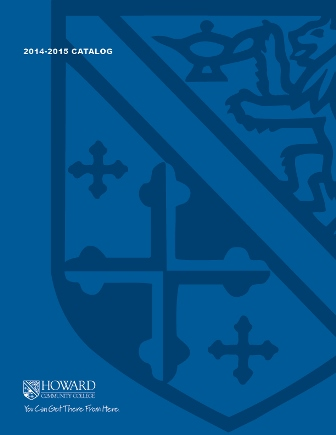2017-2018 College CatalogStudents will develop skills in initial content of both differential and integral calculus, with an emphasis on applications from business and economics. Topics include finding the limits of functions, computing derivatives of functions, analyzing graphs using the first and second derivatives, and computing definite and indefinite integrals.  Applications include supply and demand problems, marginal analysis, optimization, and consumers' and producers' surplus problems. The fundamental theorem of calculus, summations of area, partial derivatives, and the method of least squares will be used when appropriate. Students cannot receive credit for both MATH 145 and MATH 181.

3

### Prerequisite

MATH 141 or higher

3 hours weekly

### Course Objectives

1. 1. Communicate mathematical concepts related to Calculus topics using appropriate symbols,
notation, and vocabulary.
2. 2. Apply appropriate Calculus concepts, methods, and techniques to solve a variety of
application problems in business and economics such as supply and demand problems,
optimization, and marginal analysis.
3. 3. Analyze, evaluate, justify, and interpret the reasonableness of solutions to problems in
4. 4. Identify and use appropriate technologies to efficiently complete tasks that involve the solving
of a variety of mathematical problems including business and economics applications.

### Course Objectives

1. Communicate mathematical concepts related to Calculus topics using appropriate symbols,
notation, and vocabulary.

2. Apply appropriate Calculus concepts, methods, and techniques to solve a variety of
application problems in business and economics such as supply and demand problems,
optimization, and marginal analysis.

3. Analyze, evaluate, justify, and interpret the reasonableness of solutions to problems in